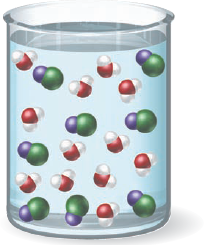×
Get Full Access to Introductory Chemistry - 5 Edition - Chapter 13 - Problem 132p
Get Full Access to Introductory Chemistry - 5 Edition - Chapter 13 - Problem 132p

×

# What is wrong with this molecular view of a sodium chloride solution? What would makeISBN: 9780321910295 34

## Solution for problem 132P Chapter 13

Introductory Chemistry | 5th Edition

• Textbook Solutions
• 2901 Step-by-step solutions solved by professors and subject experts
• Get 24/7 help from StudySoup virtual teaching assistantsIntroductory Chemistry | 5th Edition

4 5 1 347 Reviews
28
0
Problem 132P

Problem 132P

What is wrong with this molecular view of a sodium chloride solution? What would make the picture correct?Step-by-Step Solution:
Step 1 of 3

In the figure given smaller particles are water molecules and bigger particles are NaCl molecules when solid NaCl is put into water there is a clash between the attractive forces that hold Na + and Cl - particles together.and the attractive forces occuring between the particles of Na+ and Cl-and water molecule .

Hence the attractive forces occurring between the water molecules and particles of NaCl overcome the attractive forces that hold Na+ and Cl- particles together .

Reaction:

NaCl(solid) + H2O -> Na+(aqueous) + Cl-(aqueous) + H2O

Explanation:

On addition to water the Na+ section of NaCl is attracted to the oxygen side of the water molecules, while the Cl- side is attracted to the hydrogens' side of the water molecule.

This causes the sodium chloride to split in water, and the NaCl dissolves into separate Na+ and Cl- atoms. A hydration shell is formed around them which prevents Na+ and Cl- to form ionic bonds.

Thus we say the salt is dissolved.

Step 2 of 3

Step 3 of 3

##### ISBN: 9780321910295

This textbook survival guide was created for the textbook: Introductory Chemistry, edition: 5. The full step-by-step solution to problem: 132P from chapter: 13 was answered by , our top Chemistry solution expert on 05/06/17, 06:45PM. This full solution covers the following key subjects: chloride, correct, make, Molecular, picture. This expansive textbook survival guide covers 19 chapters, and 2046 solutions. Since the solution to 132P from 13 chapter was answered, more than 1264 students have viewed the full step-by-step answer. The answer to “What is wrong with this molecular view of a sodium chloride solution? What would make the picture correct?” is broken down into a number of easy to follow steps, and 18 words. Introductory Chemistry was written by and is associated to the ISBN: 9780321910295.

Unlock Textbook Solution